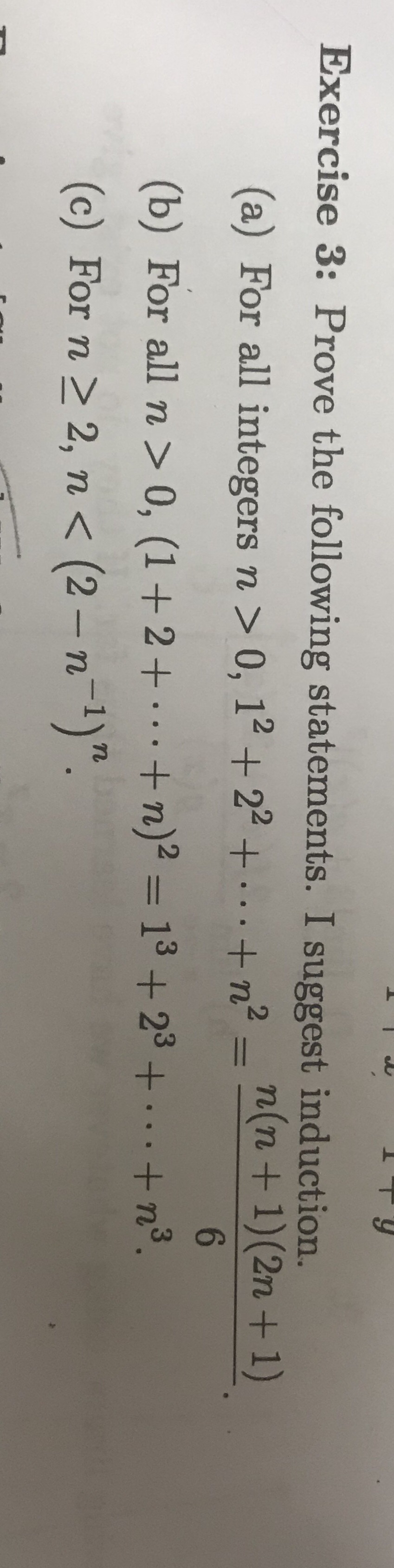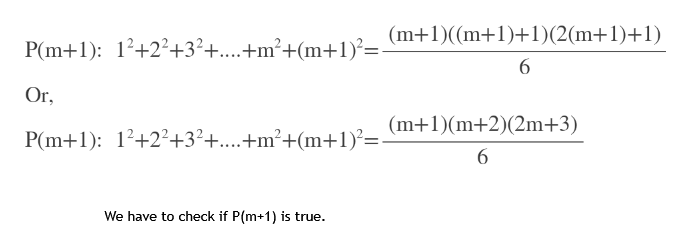# Exercise 3: Prove the following statements. I suggest induction.(a) For all integers n > 0, 12 + 22 +...+n-n(n+1) (2n + 1)6(b) For all n > 0, (1+2+.+ n)2 = 13 + 23 + .+n3.(c) For n2, n < (2 -n1)".

Question
6 views

Prove using induction:help_outlineImage TranscriptioncloseExercise 3: Prove the following statements. I suggest induction. (a) For all integers n > 0, 12 + 22 +...+n-n(n+1) (2n + 1) 6 (b) For all n > 0, (1+2+.+ n)2 = 13 + 23 + .+n3. (c) For n 2, n < (2 -n1)". fullscreen
check_circle

Step 1

We’ll answer the first question since the exact one wasn’t specified. Please submit a new question specifying the one you’d like answered.

We first check for n=1

We got 1=1, which is true

So for n=1 the statement is true.

Step 2

Let the statement is true for some m>0.

So we assume P(m) is true.

Step 3

We have to show that P(m+1) is true when...help_outlineImage TranscriptioncloseP(m+1): 12+22+3?+....+m2+(m-+1?= (m+1)((m+1)+1)(2(m+1)+1) 6 Or P(m+1) 12+22+32+...+m2+(m+1?-m+1)(m+2)(2m+3) We have to check if P(m+1) is true. fullscreen

### Want to see the full answer?

See Solution

#### Want to see this answer and more?

Solutions are written by subject experts who are available 24/7. Questions are typically answered within 1 hour.*

See Solution
*Response times may vary by subject and question.
Tagged in

### Other